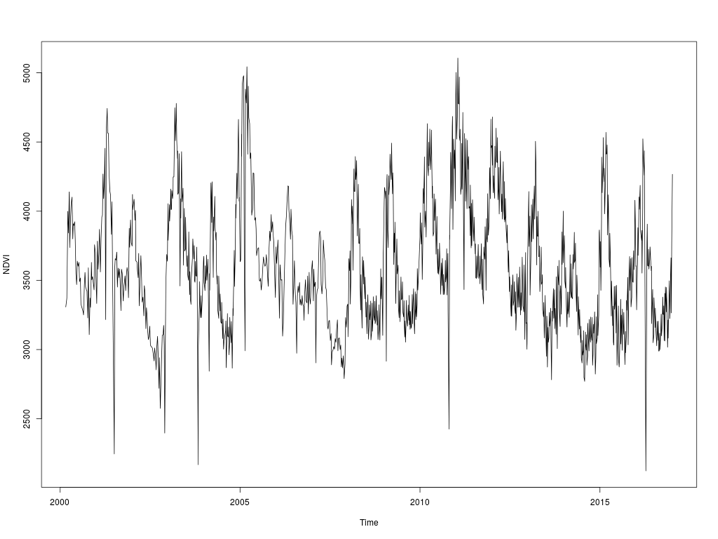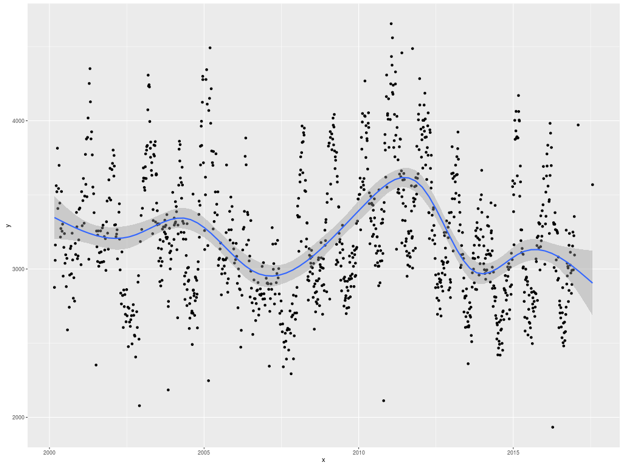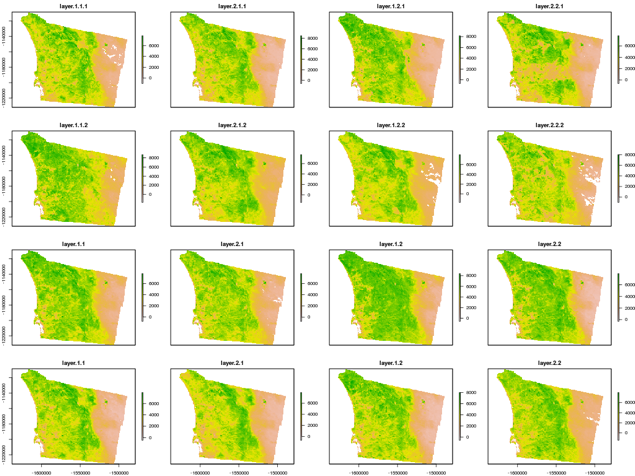This is the third and final post of the series intended to introduce PostgreSQL users to PL/R, a loadable procedural language that enables a user to write user-defined SQL functions in the R programming language. The information below provides sample use of R Functions against the NDVI dataset.

As introduced in the previous posts, the combination of PostgreSQL and R provides users with the ability to leverage the power and efficiency of PostgreSQL and the rich analytic functionality of R. When further combined with PostGIS, the geospatial extender for PostgreSQL, users can perform powerful spatial analytics within the PostgreSQL database. It is our hope that these posts will cause those building analytic applications to give PostgreSQL a second look.

The first post in this series provided users with an introduction, and discussed acquisition of administrative area boundary polygons (USA states and counties) and geocoding. The second post in this series worked through preprocessing of Normalized Difference Vegetation Index (NDVI) satellite raster data in preparation for spatial analytics.

Example Overview and Setup

This series of posts uses NDVI data from the moderate-resolution imaging spectroradiometer (MODIS) made available by the United States Geologic Survey (USGS). This data is used to demonstrate R analytic operations in PostgreSQL and PostGIS:

• Determining Average NDVI for a Particular Location Over Time
• Visualize Average NDVI for a Particular Location Over Time with ggplot
• Visualize Average NDVI for a Particular Location by Month
• Visualize Same Month Average NDVI for a Particular Location by Year

For information regarding the background for this analysis and the example set up, please take a moment to review the initial post in this series.

Analytics Operations

Having previously preprocessed and ingested the data from our example, it is now possible to perform a wide variety of R analytic functions against the data. This post works through a few examples of potential analysis as well as the resulting graphical output.

Determining Average Over Time

As an initial example of capability, we will use PL/R functions to determine the average NDVI for San Diego county as a function of time. In order to perform this analysis, first create a new function that reads the named geotiff file in which the preprocessed raster layers were stored. These layers correspond to different snapshots in time, from the year 2000 through 2016, for the NDVI values for San Diego county. The commands below create this function that is referred to as get_ndvi_mean().

This newly created function get_ndvi_mean() reads in the raster layers, and then for each layer calculates the grand average for the entire county based on the cell (pixel) level values. It combines those average county NDVI values with their corresponding average acquisition date using the method described in the previous post, and returns the results as one row per layer date and layer average pair.

The average NDVI for San Diego county as a function of time can be determined using the get_ndvi_mean() function described above by executing the following commands:

`CREATE OR REPLACE FUNCTION get_ndvi_mean  (rastfile text, OUT lyr_date date, OUT lyr_mean float8)RETURNS setof RECORD AS \$\$  library(sp)  library(raster)  # read preprocessed NDVI data  ndvi <- brick(rastfile)  # calculate layerwide-mean per layer  ndvi_mn <- cellStats(ndvi, stat = 'mean')   # read layer dates  conn <- dbConnect(dbDriver("PostgreSQL"), user="postgres", dbname="gis", host="localhost")  sql.str <- "SELECT lyr_date FROM ndvi_dates(get_robj('ndvi_dt'))"  ndvi_dt <- dbGetQuery(conn, sql.str)  # combine layer-mean with corresponding layer-date and return  return(data.frame(ndvi_dt, ndvi_mn))\$\$ LANGUAGE 'plr' STRICT;SELECT lyr_date, lyr_meanFROM get_ndvi_mean('/usr/local/bda/modis/ndvi_cooked/ndvi.tif') LIMIT 3;  lyr_date  |     lyr_mean------------+------------------ 2000-02-29 | 3307.52445819025 2000-03-09 | 3358.51562339221 2000-03-11 | 3372.62659753491(3 rows)`

A visualization operation can be performed by creating an analogous function, plot_ndvi_trend(). This plot_ndvi_trend() function operates similar to the previously demonstrated get_ndvi_mean() function, except the plot_ndvi_trend() function performs additional steps to ensure that the rows are ordered chronologically and then it plots the data, returning a png image to the SQL client in a similar way to that seen in earlier posts.

The average NDVI for San Diego county as a function of time can be determined using the plot_ndvi_trend() function described above by executing the following commands:

`CREATE OR REPLACE FUNCTION plot_ndvi_trend(rastfile text)RETURNS bytea AS \$\$  library(cairoDevice)  library(RGtk2)  library(sp)  library(raster)  # prepare in-memory buffer for the image  pixmap <- gdkPixmapNew(w=1024, h=768, depth=24); asCairoDevice(pixmap)  # read preprocessed NDVI data  ndvi <- brick(rastfile)  # calculate layerwide-mean per layer  ndvi_mn <- cellStats(ndvi, stat = 'mean')  # read layer dates  conn <- dbConnect(dbDriver("PostgreSQL"), user="postgres", dbname="gis", host="localhost")  sql.str <- "SELECT lyr_date FROM ndvi_dates(get_robj('ndvi_dt'))"  ndvi_dt <- dbGetQuery(conn, sql.str)  # create vector index sorted by date  idx <- order(ndvi_dt)  # combine sorted vectors into a data.frame  Data <- data.frame(ndvi_dt\$lyr_date[idx], ndvi_mn[idx])  # plot to the buffer  plot(Data, type ="l", xlab = "Time", ylab = "NDVI")  # capture and return the image to the client  plot_pixbuf <- gdkPixbufGetFromDrawable(NULL, pixmap, pixmap\$getColormap(),                                          0, 0, 0, 0, 500, 500)  gdkPixbufSaveToBufferv(plot_pixbuf, "png", character(0), character(0))\$buffer\$\$ LANGUAGE 'plr' STRICT;`

The average NDVI for San Diego county as a function of time determined by the plot_ndvi_trend() function can be returned as a png image to the SQL Client by executing the following commands:

`SELECT plr_get_raw(plot_ndvi_trend('/usr/local/bda/modis/ndvi_cooked/ndvi.tif'));`

The graphical output for average NDVI over time is provided in Figure 1 below.

Figure 1: Average NDVI Over Time Determined by plot_ndvi_trend() FunctionVisualize Average over Time with ggplot

The average NDVI for San Diego county as a function of time can also be visualized with an alternative plotting function called ggplot(). The following pure R code will use this alternative plotting method to produce a png image file:

`library(sp)library(raster)library(ggplot2)library(RPostgreSQL)# might not be required depending on client setupSys.setenv("DISPLAY"=":0.0")# read preprocessed NDVI datandvi <- brick(rastfile)# calculate layerwide-mean per layerndvi_mn <- cellStats(ndvi, stat = 'mean')# read layer datesconn <- dbConnect(dbDriver("PostgreSQL"), user="postgres", dbname="gis", host="localhost")sql.str <- "SELECT lyr_date FROM ndvi_dates(get_robj('ndvi_dt'))"ndvi_dt <- dbGetQuery(conn, sql.str)# create vector index sorted by dateidx <- order(ndvi_dt)# combine sorted vectors into a data.frameData <- data.frame(ndvi_dt\$lyr_date[idx], ndvi_mn[idx])# use ggplot to create png file with the plot outputpng("/tmp/ndvi_trend_gg.png", width = 1024, height = 768)ggplot(Data, aes(x,y)) + geom_point() + geom_smooth()# flush the output to filedev.off()`

The graphical output for average NDVI for San Diego county over time visualized using ggplot is provided in Figure 2 below.

Figure 2: Average NDVI Over Time Visualized Using ggplotNote that the geom_smooth() argument to ggplot provided a nice regression line with confidence bands. Various smoothing methods are available, but here we have allowed ggplot to select the default. This addition aids the eye in spotting trends in the data.

Visualize Average by Month

Using R commands, it is possible to create a visualization of the average NDVI for San Diego county by month for a given year. This visualization can be generated by executing the following commands:

`plot_ndvi_year <- function(rastfile, layeryr, plotfile) {  library(sp)  library(raster)  library(RPostgreSQL)  Sys.setenv("DISPLAY"=":0.0")  # required in R, noop in PL/R  conn <- dbConnect(dbDriver("PostgreSQL"), user="postgres", dbname="gis", host="localhost")  # plot to file if destination filename given  if (plotfile != "") png(plotfile, width = 1024, height = 768)  # read the dates  conn <- dbConnect(dbDriver("PostgreSQL"), user="postgres", dbname="gis", host="localhost")  sql.str <- "SELECT lyr_date FROM ndvi_dates(get_robj('ndvi_dt'))"  ndvi_dt <- dbGetQuery(conn, sql.str)  # read and plot requested raster layer  ndvi <- brick(rastfile)  ndvi_rast <- raster()  # for each month and selected year, do pixel-wise aggregation to get one layer per month  for (i in 1:12) {    fmt_str <- paste(layeryr, sprintf("%02d", i), sep = "-")    ndvi_layer <- mean(ndvi[[which(format(ndvi_dt, "%Y-%m") == fmt_str)]], na.rm = TRUE)    ndvi_rast <- addLayer(ndvi_rast, ndvi_layer)  }  # plot and return  plot(ndvi_rast)  if (plotfile != "") dev.off()}plot_ndvi_year("/usr/local/bda/modis/ndvi_cooked/ndvi.tif", 2011, "/tmp/ndvi-2011.png")`

It is worth noting that in this case, rather than averaging an entire raster layer to provide one NDVI value for the county, the commands are averaging pixel-by-pixel across multiple raster layers per month, in order to provide a composite image representing the given month. The visual output for average NDVI by month for the year 2011 is provided in Figure 8 below.

Figure 3: Visualization Average NDVI for San Diego County by MonthVisualize Same Month Average by Year

Using similar R commands, it is possible to create a visualization of the average NDVI for San Diego county for a given month, over a series of years.

`plot_ndvi_month <- function(rastfile, layermonth, firstyr, lastyr, plotfile) {  library(sp)  library(raster)  library(RPostgreSQL)  Sys.setenv("DISPLAY"=":0.0")  # required in R, noop in PL/R  conn <- dbConnect(dbDriver("PostgreSQL"), user="postgres", dbname="gis", host="localhost")  # plot to file if destination filename given  if (plotfile != "") png(plotfile, width = 1024, height = 768)  # read the dates  conn <- dbConnect(dbDriver("PostgreSQL"), user="postgres", dbname="gis", host="localhost")  sql.str <- "SELECT lyr_date FROM ndvi_dates(get_robj('ndvi_dt'))"  ndvi_dt <- dbGetQuery(conn, sql.str)  # read and plot requested raster layer  ndvi <- brick(rastfile)  ndvi_rast <- raster()  # for each year and selected month, do pixel-wise aggregation to get one layer per month  for (i in firstyr:lastyr) {    fmt_str <- paste(i, sprintf("%02d", layermonth), sep = "-")    ndvi_layer <- mean(ndvi[[which(format(ndvi_dt, "%Y-%m") == fmt_str)]], na.rm = TRUE)    ndvi_rast <- addLayer(ndvi_rast, ndvi_layer)  }   plot(ndvi_rast)  if (plotfile != "") dev.off()}`

The visual output for average NDVI for San Diego county for the month of January from 2001 to 2016 can be generated using the following command and is provided in Figure 4 below.

`plot_ndvi_year("/usr/local/bda/modis/ndvi_cooked/ndvi.tif", 1, 2001, 2016, "/tmp/ndvi-1.png")`

Figure 4: Visualization of Average NDVI for San Diego County by MonthConclusion

While PostgreSQL is not necessarily known for use with analytic applications, the combination of PostgreSQL, PostGIS, PL/R and R provide PostgreSQL users with the combination of the power and efficiency of PostgresSQL and the rich analytic functionality of R. When further combined with PostGIS, the geospatial extender for PostgreSQL, users can perform powerful spatial analytics within the PostgreSQL database.